Geometry 6-5 Conditions for Rhombuses, Rectangles, and Squares
starstarstarstarstarstarstarstarstarstar
by Matthew Richardson
| 29 Questions
Note from the author:
A complete formative lesson with embedded slideshow, mini lecture screencasts, checks for understanding, practice items, mixed review, and reflection. I create these assignments to supplement each lesson of Pearson's Common Core Edition Algebra 1, Algebra 2, and Geometry courses. See also mathquest.net and twitter.com/mathquestEDU.
The outlined content above was added from outside of Formative.
The outlined content above was added from outside of Formative.1
1
10 pts
Solve It! Which vertices form a square? Select all that apply.
AFEO
BCDG
GDHO
GJFL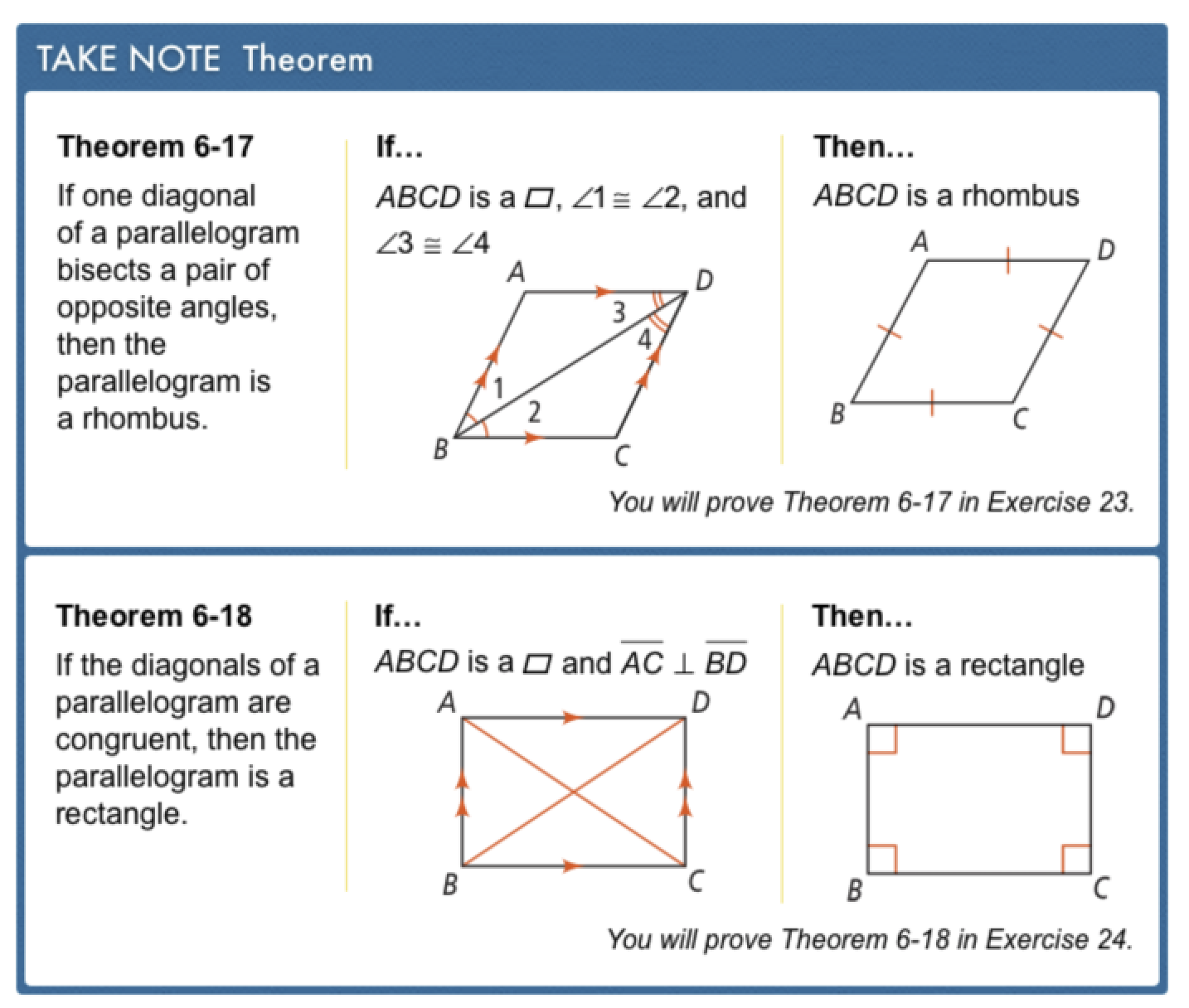The outlined content above was added from outside of Formative.
2
10 pts
Problem 1 Got It? A parallelogram has angle measures of 20, 160, 20, and 160. Can it be a rhombus, a rectangle, or a square?
Rhombus
Rectangle
Square
3
10 pts
Problem 1 Got It? Explain your response on the previous item.
4
10 pts
Problem 1 Got It? Reasoning: Suppose the diagonals of a quadrilateral bisect each other. Can you conclude that it is a rhombus, a rectangle, or a square?
Rhombus
Rectangle
Square
The quadrilateral is none of these.
There is not enough information to make a conclusion.
5
10 pts
Problem 1 Got It? Explain your response on the previous item.
The outlined content above was added from outside of Formative.6
6
10 pts
Problem 2 Got It?
A
B
C
D
The outlined content above was added from outside of Formative.7
7
10 pts
Problem 3 Got It? Can you adapt the method in Problem 3 slightly to stake off a square play area? Explain.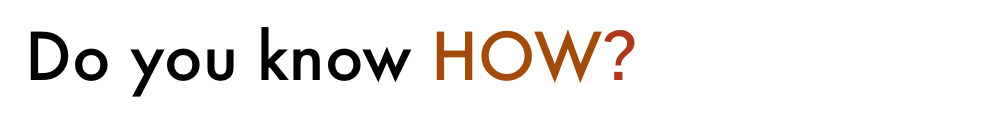8
9
10
11
8
10 pts
Based on the given information, what are the possible classifications for the parallelogram?
Select all that apply.
Rhombus
Rectangle
Square
None of these
9
10 pts
Explain your response to the previous item.
10
10 pts
Based on the given information, what are the possible classifications of the parallelogram?
Select all that apply.
Rhombus
Rectangle
Square
None of these
11
10 pts
Explain your response to the previous item.12
12
10 pts
A
B
C
D13
13
10 pts
A
B
C
D14
10 pts
Identify the special parallelograms that have each property.
• Rhombuses
• Rectangles
• Squares
• Diagonals are perpendicular
• Diagonals are congruent
• Diagonals are angle bisectors
• Diagonals bisect each other
• Diagonals are perpendicular bisectors of each other
15
10 pts
Error Analysis: Your friend says, "A parallelogram with perpendicular diagonals is a rectangle." What is your friend's error? Explain.
16
10 pts
Reasoning: Complete the following steps on the canvas.
1. Draw a circle and two of its diameters.
2. Connect the endpoints of the diameters to form a quadrilateral.
17
10 pts
Reasoning: Which types of quadrilaterals are possible in the previous item? Select all that apply.
Rhombuses
Rectangles
Squares
None of these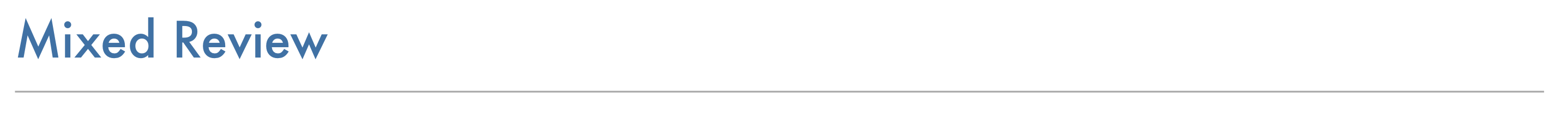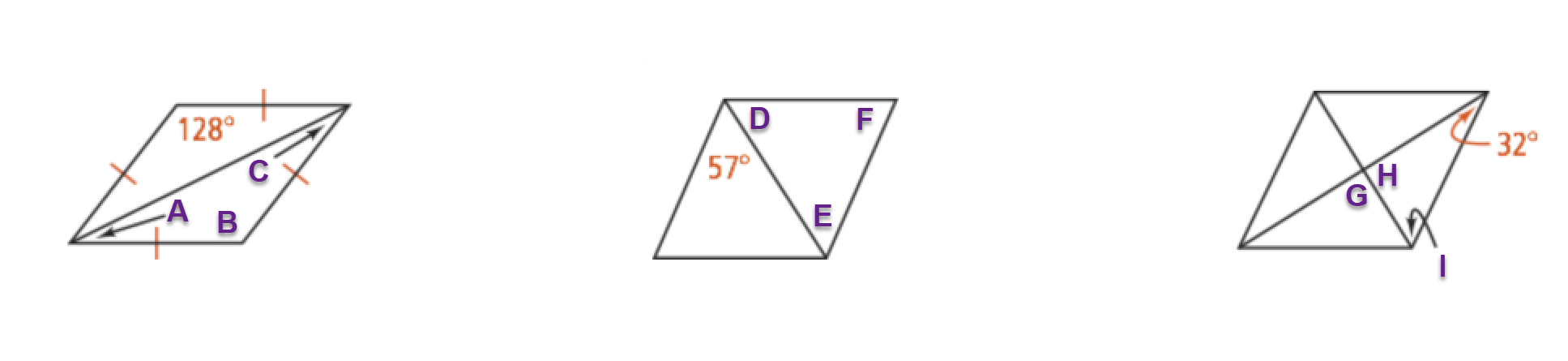18
10 pts
Review Lesson 6-4: Use the parallelograms in the diagrams above to match the measures with their angles.

(Tip: You may need to use the down arrow key to drag items to the lowest angle measures.)
• 58
• 26
• 90
• 57
• 128
• 66
• ∠A
• ∠B
• ∠C
• ∠D
• ∠E
• ∠F
• ∠G
• ∠H
• ∠I
19
10 pts
Review Lesson 2-3: Write the two conditionals as a biconditional.

If a parallelogram is a rhombus, then its diagonals are perpendicular.
If the diagonals of a parallelogram are perpendicular, then the parallelogram is a rhombus.
20
10 pts
Review Lesson 2-3: Write the two conditionals as a biconditional.

If a parallelogram is a rectangle, then its diagonals are congruent.
If the diagonals of a parallelogram are congruent, then the parallelogram is a rectangle.
Review Lesson 1-321
22
23
24
21
10 pts
Find the value of a.
Enter only a number.
22
10 pts
Find the value of b.
Enter only a number.
23
10 pts
Find the value of m.
Enter only a number.
24
10 pts
Find the value of n.
Enter only a number.25
10 pts
Vocabulary Review: Cross out the figure that is NOT a quadrilateral.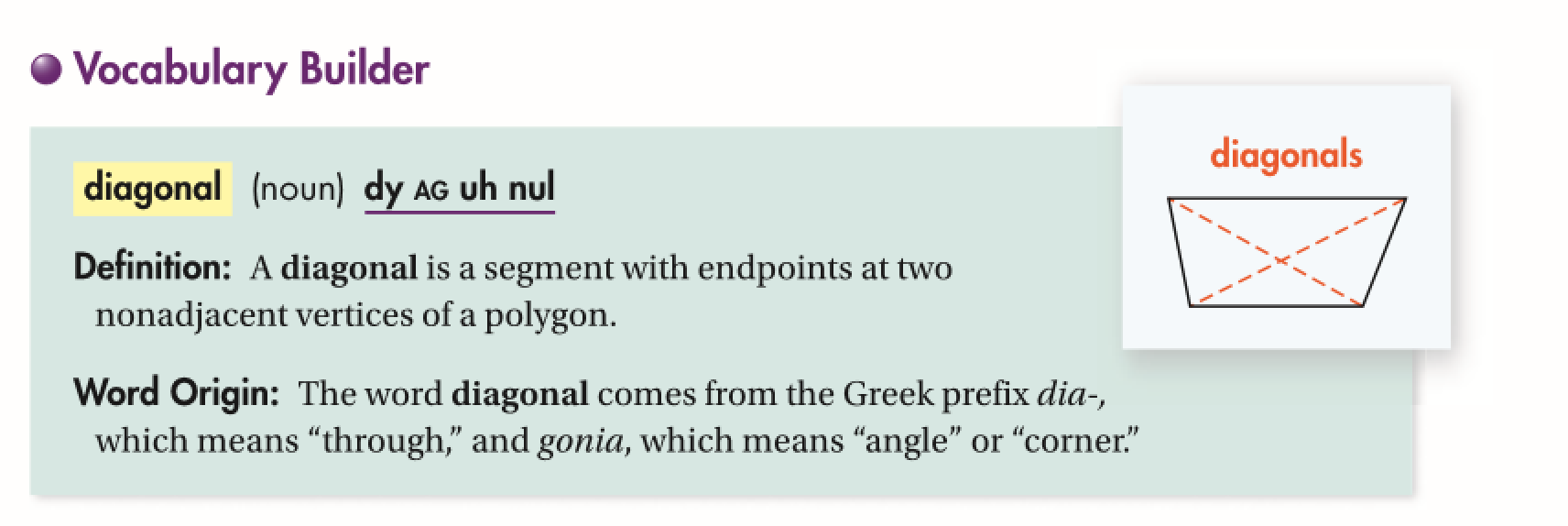26
10 pts
Use Your Vocabulary: Which polygon has no diagonal ?
triangle
pentagon
hexagon
27
10 pts
Use Your Vocabulary: Which polygon has two diagonals ?
triangle